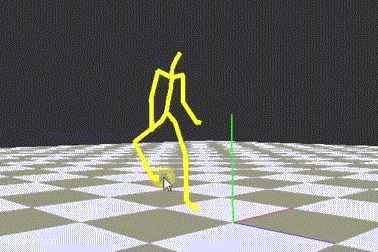# Inverse Kinematics## Overview

In contract to Forward Kinematics, which computes the end-effectors through the parameters of segments, Inverse kinematics would derive approximate parameters for segments from a decided end-effector.

This project used a framework developed with C++ and OpenGL by GPLab, NCTU.

## Inverse-Jacobian Method

Inverse-Jacobian method is a rather simple and effective way of implementing Inverse Kinematics. It models the forward kinematics equation using a Taylor series expansion, which is simpler to invert and solve than the original system.

This technique computes an estimate set of parameters of segements to minimize the error to end-effector. It computes iteratively to adjust until the solution set of parameters is close enough to the end-effector. Applying this method may result in very rough estimate of the decided end-effector.

Here I set the computing step of IK to 30.0, and error factor is 0.0001. The big step is to make the process more real-time, while the error factor is a reasonable value for finding the good enough result.

## Results

Although the performance is acceptable, the poses this method computed are not spontaneous enough. In this case, I used a human skeleton, but some of the poses with the limbs twisting to weird angle to match the end-effector were not normal at all. Therefore, for more spontaneous results, it needs some improvements, such as feature-based IK or style-based IK.

Tags:

Categories: